﻿ 融合小波包分解和CSA的电路故障诊断方法

# 融合小波包分解和CSA的电路故障诊断方法A Circuit Fault Diagnosis Method by Fusing Wavelet Packet Decomposition and CSA

Abstract: The basic characteristics of analog circuit make it very difficult to diagnose. A circuit fault diagnosis method by fusing wavelet packet decomposition and CSA is proposed to this problem. Firstly, wavelet packet is introduced to decompose, reconstruct and analyze kinds of fault voltage signals output by analog circuit; the frequency band energy of the corresponding spectrum is obtained as a fault characteristic sample, including training samples and test samples. Then the training samples were studied by using the CSA, and the optimal cluster center was obtained. Finally, the fault is classified according to the Euclidean distance between the test sample and the cluster center, and the fault element localization of the analog circuit is realized. The experimental results show that the method has higher diagnostic accuracy and shorter convergence time.

1. 引言

2. 小波包分析

$\left\{\begin{array}{l}{U}_{j}^{0}={V}_{j}\\ {U}_{j}^{1}={W}_{j}\end{array},\text{\hspace{0.17em}}\text{\hspace{0.17em}}j\in Z$ (1)

${\omega }_{2n}\left(t\right)=\sqrt{2}{\sum }_{k}h\left(k\right){\omega }_{n}\left(2t-k\right)$ (2)

${\omega }_{2n+1}\left(t\right)=\sqrt{2}{\sum }_{k}g\left(k\right){\omega }_{n}\left(2t-k\right)$ (3)

${V}_{i+1}={V}_{j}\oplus {W}_{j}$ (4)

$\left\{\begin{array}{l}{U}_{j+1}^{0}={U}_{j}^{0}\oplus {U}_{j}^{1},\text{\hspace{0.17em}}\text{\hspace{0.17em}}j\in Z\\ {U}_{j+1}^{n}={U}_{j}^{2n}\oplus {U}_{j}^{2n+1},\text{\hspace{0.17em}}\text{\hspace{0.17em}}j\in Z\end{array}$ (5)

$\left\{{d}_{l}^{j+1,n}\right\}$$\left\{{d}_{l}^{j,2n}\right\}$$\left\{{d}_{l}^{j,2n+1}\right\}$ 的公式

${d}_{l}^{j,2n}={\sum }_{k}{h}_{k}-2l{d}_{k}^{j+1,n}$ (6)

${d}_{l}^{j,2n+1}={\sum }_{k}{g}_{k}-2l{d}_{k}^{j+1,n}$ (7)

$\left\{{d}_{l}^{j,2n}\right\}$$\left\{{d}_{l}^{j,2n+1}\right\}$$\left\{{d}_{l}^{j+1,n}\right\}$ 的公式

${d}_{l}^{j+1,n}={\sum }_{k}\left({p}_{l}-2k{d}_{k}^{j,2n}+{q}_{l}-2k{d}_{k}^{i,2n+1}\right)$ (8)

$S=AA{A}_{3}+DA{A}_{3}+AD{A}_{3}+DD{A}_{3}+AA{D}_{3}+DA{D}_{3}+AD{D}_{3}+DD{D}_{3}$

3. 克隆选择算法

1) 高频变异：变异率与亲和力成反比，变异函数定义为：

$MAb11_{V}^{\prime }=MAb11_V+\beta \left(Ao\left(i\right)-MAb11_V\right)×N\left(0,1\right)$ (9)

${f}_{i}=\frac{1}{1+{D}_{i}}$ (10)

${D}_{i}=‖MAb_T\left(i\right)-Ao\left(i\right)‖$ (11)

2) 克隆删除：免疫细胞经过变异后产生的低亲和力的免疫细胞，因得不到与抗原结合的机会而死亡。

3) 克隆增值：根据免疫细胞与抗原的亲和力大小对免疫细胞进行克隆繁殖，亲和力越高，克隆繁殖的机会越大，克隆的数目越多。克隆数目定义为：Figure 1. Schematic diagram of wavelet packet decomposition structure

${N}_{c}=round\left({f}_{i}×K\right)$ (12)

4. 模拟电路故障诊断

1) 将所获得的故障样本归一化后，一部分作为训练样本 $MAb_X\left(j\right)\left(j=1,2,\cdots ,9\right)$ ，一部分作为测试样本 $MAb_T\left(j\right)\left(j=1,2,\cdots ,9\right)$

2) 删除亲和力最低的10%的免疫细胞，选择亲和力为前90%的免疫细胞进行训练。

3) 根据式(12)确定抗体克隆数目并克隆选择出的免疫细胞，其中K取10。

4) 根据式(9)对克隆的个体进行变异。

5) 在变异后的免疫细胞中选择亲和力前10%的免疫细胞进入记忆细胞中和原来的记忆细胞集组成新的记忆细胞集。

6) 对抗体的聚类中心进行更新。直到满足终止条件，退出并保存抗体的聚类中心，否则转向步骤(2)。

7) 根据式(11)计算抗原与聚类中心的欧氏距离，实现模拟电路故障定位。

5. 诊断实例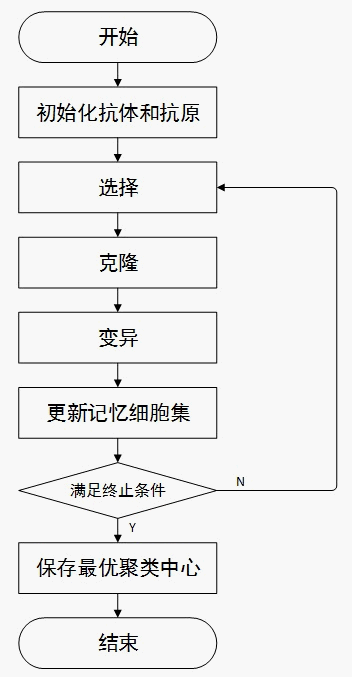Figure 2. Flow chart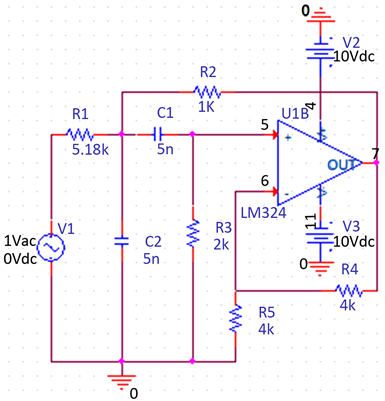Figure 3. Sallen-key band pass filter circuitTable 1. Sallen-key band pass filter circuit fault mode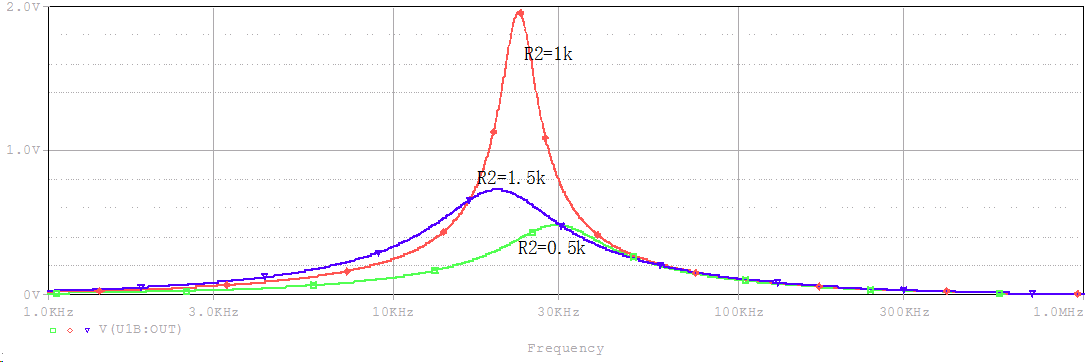Figure 4. The normal state compared with R2 (+50%) and R2 (−50%)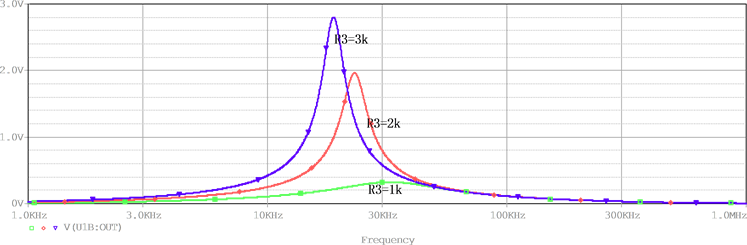Figure 5. The normal state compared with R3 (+50%) and R3 (−50%)Figure 6. The normal state compared with C1 (+50%) and C1 (−50%)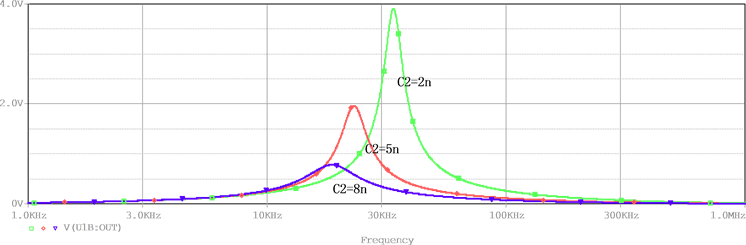Figure 7. The normal state compared with C2 (+50%) and C2 (−50%)Table 3. Comparison of simulation results

6. 结语

 丁伟聪, 李志华, 裴洁才. 基于覆盖算法的模拟电路故障诊断方法[J]. 计算机与现代化, 2017(1): 36-40.

 秦亮, 王朕. 一种基于免疫克隆聚类的模拟电路故障诊断方法[J]. 仪表技术, 2017(5): 22-26.

 周启忠, 谢永乐. 基于矩阵扰动分析的模拟电路故障诊断方法[J]. 西南交通大学学报, 2017(2): 369-378.

 蔡鑫, 南新元, 高丙朋. ICS优化SVM在模拟电路故障诊断中的应用[J]. 科技通报, 2017(4): 79-82.

 苏宝林, 李震. 基于最大异类距离和正则极端学习机的模拟电路在线故障诊断[J]. 仪表技术与传感器, 2017(2): 116-121.

 Khanali, M., Hayati-Soloot, A. and Hoidalen, H.K. (2017) Study on Locating Transformer Internal Faults Using Sweep Frequency Response Analysis. Electric Power Systems Research, 145, 55-62.
https://doi.org/10.1016/j.epsr.2016.11.016

 邓勇, 于晨松, 文浩. 基于倒谱和决策树的模拟电路故障诊断[J]. 电子测量与仪器学报, 2017(3): 430-435.

 陈博文, 李志华, 黄颖. 点对主分量分析算法的模拟电路故障诊断研究[J]. 电子设计与工程, 2017(7): 126-129.

 颜学龙, 丁鹏, 马峻. 基于狼群算法的RBF神经网络模拟电路故障诊断[J]. 计算机工程与应用, 2017, 53(19): 152-156.

 禹旺兵, 彭良玉, 禹恒州. 基于小波分析和人工免疫算法的模拟电路故障诊断[J]. 自动化技术, 2006(19): 76-78.

 Shang, R.H., Du, B.Q. and Ma, H.N. (2016) Immune Clonal Algorithm Based on Directed Evolution for Mul-ti-Objective Capacitated Arc Routing Problem. Applied Soft Computing, 49, 748-758.
https://doi.org/10.1016/j.asoc.2016.09.005

 韩富春, 高文军, 廉建鑫. 基于免疫优化多分类SVM的变压器故障诊断新方法[J]. 电力系统保护与控制, 2012(2): 106-110.

 彭良玉, 禹旺兵. 基于小波分析和克隆选择算法的模拟电路故障诊断[J]. 电工技术学报, 2007(6): 12-16.

 Aminian, F. and Aminian, M. (2001) Fault Diagnosis of Nonlinear Analog Circuits using Neural Networks with Wavelet and Fourier Transforms as Preprocessors. Journal of Electronic Testing, 17, 471-481.
https://doi.org/10.1023/A:1012864504306

 宝石, 许军. 基于信息融合的模拟电路故障的特征提取与融合方法[J]. 计算机测量与控制, 2017(8): 1-4.

Top